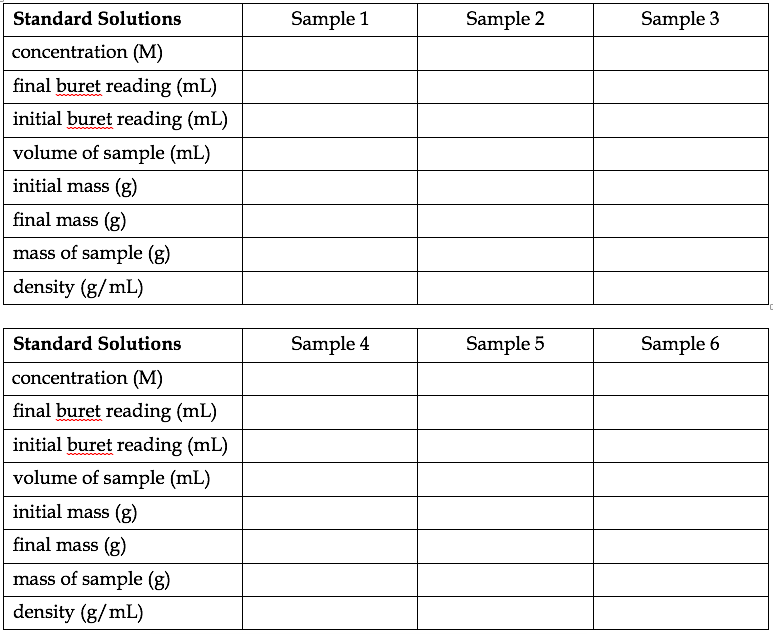# 2: Determination of the Molar Concentration of a Saline Solution

## INTRODUCTION

At a given temperature, the density of an aqueous solution of sodium chloride, sometimes called “saline,” is a function of concentration. As the concentration of NaCl increases, the density of the solution increases in a fairly linear manner. In this experiment, you will analyze a series of standard saline solutions of known concentration. You will construct a standard curve by plotting density vs. concentration in a spreadsheet, which will give you an equation that relates the two parameters. This equation will allow you to determine the molar concentration of your unknown solution from the previous lab period.

## OBJECTIVES

1. To construct a standard curve showing the relationship between two properties.

2. To determine the molar concentration of an unknown solution.

## PROCEDURE

1. Record the concentrations of the given standard solutions.

2. Record the mass of an empty 125-mL Erlenmeyer flask (it does not have to be dry).

3. Carefully record the initial buret reading for one of the solutions, then drain about 20 mL of the solution into your weighed flask. Record the final buret reading (each reading needs to be precise but do not worry about being exact).

4. Record the mass of the flask with the solution, then empty the flask.

5. Repeat steps 2–4 using each of the standard solutions, so that you can determine the density of all six solutions.

### CALCULATIONS

1. Calculate the density of each solution. The density should increase as the concentration increases. If your results do not follow this pattern, double-check your calculations and consult with your instructor.

2. Once you have the density for each of the six solutions, you will use a spreadsheet program (e.g. Excel) to plot density vs. concentration. Refer to the separate guidelines or ask your instructor for help when making this plot.

3. The trendline that comes from your plot will be a linear equation that you can use to determine the molar concentration of the unknown solution from the previous lab session. The equation will have the form y = mx + b, where y is the density, m is the slope of the line, x is the concentration, and b is the y-intercept (what do you expect the value of this intercept to be?). Insert the average density of your unknown solution into this equation and you will determine its concentration.

As a guide, you should have data tables in your notebook that resemble the ones below. DO NOT write data on this page! Remember that it is important to record each measurement (e.g. individual buret readings), not just the results of the measurements.A copy of your printed graph should be firmly attached to your notebook. Your conclusion should state the mathematical relationship between density and concentration, and should include the calculated result for the molar concentration of your unknown solution from Experiment A (with its ID code).